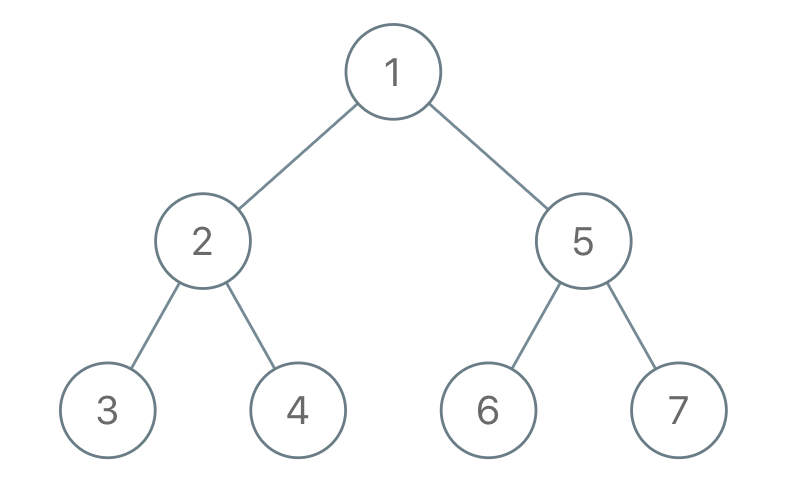# 1028. Recover a Tree From Preorder Traversal

We run a preorder depth first search on the `root`of a binary tree.

At each node in this traversal, we output `D` dashes (where `D` is the  depth  of this node), then we output the value of this node.   (If the depth of a node is`D`, the depth of its immediate child is `D+1`.  The depth of the root node is `0`.)

If a node has only one child, that child is guaranteed to be the left child.

Given the output `S` of this traversal, recover the tree and return its `root`.

Example 1:``````Input: "1-2--3--4-5--6--7"
Output: [1,2,5,3,4,6,7]
``````

Example 2:``````Input: "1-2--3---4-5--6---7"
Output: [1,2,5,3,null,6,null,4,null,7]
``````

Example 3:``````Input: "1-401--349---90--88"
Output: [1,401,null,349,88,90]
``````

Note:

• The number of nodes in the original tree is between `1` and `1000`.
• Each node will have a value between `1` and `10^9`.

``````class Solution {
public:
TreeNode* recoverFromPreorder(string S) {
vector<TreeNode*> st;
int i = 0, level = 0, val = 0, n = S.size();
while (i < n) {
for (level = 0; i < n && S[i] == '-'; ++i) {
++level;
}
for (val = 0; i < n && S[i] != '-'; ++i) {
val = 10 * val + (S[i] - '0');
}
TreeNode *node = new TreeNode(val);
while (st.size() > level) st.pop_back();
if (!st.empty()) {
if (!st.back()->left) st.back()->left = node;
else st.back()->right = node;
}
st.push_back(node);
}
return st;
}
};
``````

``````class Solution {
public:
TreeNode* recoverFromPreorder(string S) {
int cur = 0;
return helper(S, cur, 0);
}
TreeNode* helper(string& S, int& cur, int level) {
int cnt = 0, n = S.size(), val = 0;
while (cur + cnt < n && S[cur + cnt] == '-') ++cnt;
if (cnt != level) return nullptr;
cur += cnt;
for (; cur < n && S[cur] != '-'; ++cur) {
val = 10 * val + (S[cur] - '0');
}
TreeNode *node = new TreeNode(val);
node->left = helper(S, cur, level + 1);
node->right = helper(S, cur, level + 1);
return node;
}
};
``````

Github 同步地址:

https://github.com/grandyang/leetcode/issues/1028

Construct Binary Tree from Inorder and Postorder Traversal

Construct Binary Tree from Preorder and Inorder Traversal

https://leetcode.com/problems/recover-a-tree-from-preorder-traversal/

https://leetcode.com/problems/recover-a-tree-from-preorder-traversal/discuss/274656/Java-recursive-solution.

https://leetcode.com/problems/recover-a-tree-from-preorder-traversal/discuss/274621/JavaC%2B%2BPython-Iterative-Stack-Solution

LeetCode All in One 题目讲解汇总(持续更新中…)

 微信打赏Venmo 打赏×

Help us with donation